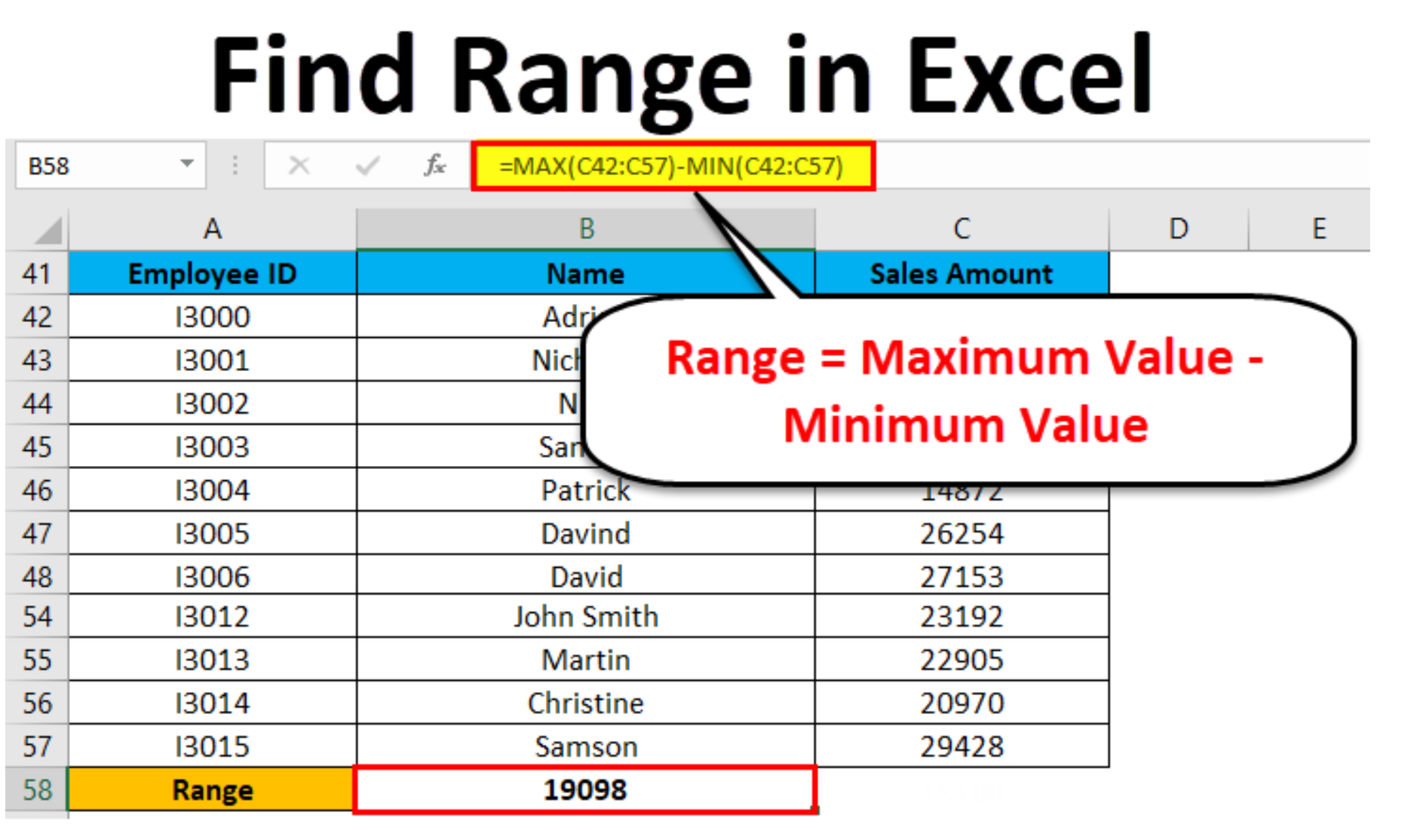# How to Calculate Range in Excel

When you come across the word range, it simply means the difference between the minimum and maximum values in a dataset. When working with Excel, you can calculate the range of the functions MIN and MAX.## Meaning of Range

Range is the difference between minimum and maximum. It tells you the spread of values in a data set. This is the simple definition:

Range = maximum value – minimum value.

In a situation where you have a set of data like 4, 2, 5, 8, 12, 15, the range would be the highest number which is (15) minus the lowest number.

So Range =15-2 = 13

With this example, you can tell that the data set spans 13 numbers. If it is a box and whisker plot, the ends of the whiskers offer you a visual indication of the range. This is because they mark the minimum and maximum values. A large range will typically suggest a wide spread of results, while a small range suggests data which is closely centered around a specific value

## Minimum and Maximum Formulas

Even though range is commonly used, there is no built-in RANGE function in Excel. However, you can use this formula in calculating range. To find a range in Excel you have to obtain the minimum and maximum values of the data set. To find the minimum,

• Type ‘=MN ‘ into a cell
• You can either double click where you find ‘MN’ in the drop-down list of type a ‘(‘ to start inside the parentheses
• Enter the column letter as well as row number of the first data point.
• If your data is in a single row or column
• Type a column followed by the letter and number which corresponds to the last data point and thereafter close the parentheses in order to return to the minimum value.
• Alternatively, you can click the appropriate cell after opening the parentheses and then holding down ‘Shift’. Then click the cell with the last data point before you close the parentheses.

Where the data is spread out irregularly, you can have each cell specified individually, separating each one with a comma.

You can use the MAX function in finding the maximum value from the data set by typing ‘MAX’ into a cell, thereafter repeat the same process as that of the ‘MIN’ function.

## Range in Excel in Steps

To easily use Excel for range, calculate in steps:

• Start by placing the minimum value in a cell
• Place the maximum value in another cell
• In another cell, type ‘=F1-E1’ to find the range.
• This will intimate Excel to subtract the minimum in cell E1 from the maximum in cell F1. This will give you the range.

## How to Calculate Range in One Step

Alternatively, you can use the formulas stated above for minimum and maximum to Calculate the Range with the use of Excel in one step.